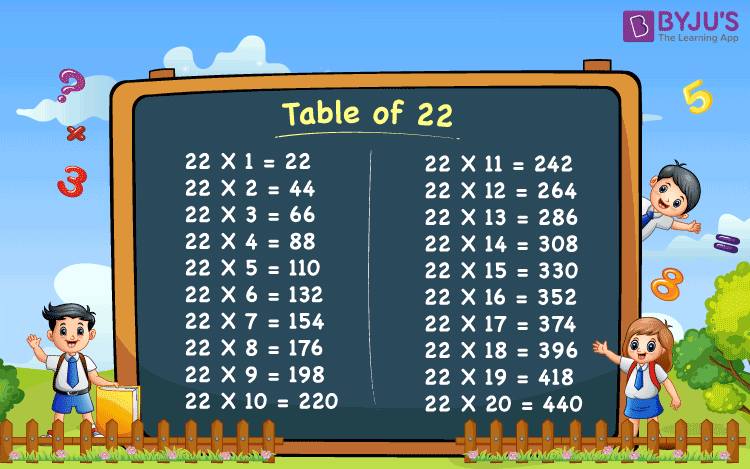# Table of 22

In this, we will read and memorise the table of 22. You can also download the 22 times table image for further reference. Multiplication tables help us to solve mathematical problems easily and quickly. These tables are necessary for your primary classes, such as Class 1 to Class 5. Students of the primary classes can solve arithmetic problems quickly in exams by memorising the tables from 1 to 30.

Download the 22 Times Table PDF here; it will help memorise the table easily.

## Table of 22 Chart## 22 Times table

Go through the 22 times table from 1 to 20 given below:

 22 X 1 = 22 22 X 2 = 44 22 X 3 = 66 22 X 4 = 88 22 X 5 = 110 22 X 6 = 132 22 X 7 = 154 22 X 8 = 176 22 X 9 = 198 22 X 10 = 220 22 X 11 = 242 22 X 12 = 264 22 X 13 = 286 22 X 14 = 308 22 X 15 = 330 22 X 16 = 352 22 X 17 = 374 22 X 18 = 396 22 X 19 = 418 22 X 20 = 440

Repeatedly adding by 22’s gives the table of 22.

Also, check:

Tables from 21 to 30

Tables 11 to 30

### How to Read 22 Times Table

Read the twenty-two times table from 1 to 10 given below.

• One time twenty-two is twenty-two.
• Two times twenty-two is forty-four.
• Three times twenty-two is sixty-six.
• Four times twenty-two is eighty-eight.
• Five times twenty-two is one hundred and ten.
• Six times twenty-two is one hundred and thirty-two.
• Seven times twenty-two is one hundred and fifty-four.
• Eight times twenty-two is one hundred and seventy-six.
• Nine times twenty-two is one hundred and ninety-eight.
• Ten times twenty-two is two hundred and twenty.

### Examples

(i) When 2 packs each having 22 pastel colours.

By repeated addition we can show 22 + 22 = 44

Then, twenty-two 2 times are

22 × 2 = 44

Therefore, there are 44 pastel colours.

(ii) When 3 bunches of 22 bananas each.

By repeated addition we can show 22 + 22 + 22 =66 Then, twenty-two 3 times are;

22 × 3 = 66

Therefore, there are 66 bananas.

(iii) When 4 bunches of 22 bananas each.

By repeated addition we can show 22 + 22 + 22 +22 = 88

Then, twenty-two 4 times are:

22 × 4 = 88

Therefore, there are 88 bananas.

(iv) When 5 bunches of 22 bananas each.

By repeated addition we can show 22 + 22 + 22 + 22 +22 = 110

Then, twenty-two 5 times are:

22 × 5 = 110

Therefore, there are 110 bananas.

(v) When 6 bunches of 22 bananas each.

By repeated addition we can show 22 + 22 + 22 + 22 + 22 + 22 = 132

Then, twenty-two 6 times are:

22 × 6 = 132

Therefore, there are 132 bananas.

(vi)When 7 bunches of 22 bananas each.

By repeated addition we can show 22 + 22 + 22 + 22 + 22 + 22 +22 = 154

Then, twenty-two 7 times are:

22 × 7 = 154

Therefore, there are 154 bananas.

(vii) When 8 bunches of 22 bananas each.

By repeated addition we can show 22 + 22 + 22 + 22 + 22 + 22 + 22 + 22 = 176

Then, twenty-two 8 times are:

22 × 8 = 176

Therefore, there are 176 bananas.

(viii) When 9 bunches of 22 bananas each.

By repeated addition we can show 22 + 22 + 22 + 22 + 22 + 22 + 22 + 22 +22 = 198

Then, twenty-two 9 times are:

22 × 9 = 198

Therefore, there are 198 bananas.

(ix) When 10 bunches of 22 bananas each.

By repeated addition we can show 22 + 22 + 22 + 22 + 22 + 22 + 22 + 22 +22 +22 = 220

Then, twenty-two 10 times are:

22 × 10 = 220

Therefore, there are 220 bananas.

Multiplication of numbers is important to understand for everyday life application of numbers. (Maths) Tables play a main role in it.

Forward counting : 22, 44, 66, 88,110,132,154,176,198, 220, 242, 264, 286, 308, 330, 352, 374, 418, 440, 462.

Backward counting : 462, 440, 418, 374, 352, 330, 308, 286, 264, 242, 220, 198, 176, 154, 132, 110, 88, 66 ,44, 22.

Test your knowledge on Table Of 22